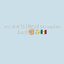Mathematics 90 OnlineOpenStudy (connor):

A man built a house costing 9 times as much as the lot on which it was built. If the lot cost \$5000 less than 1/6 the cost of the house. What was the cost of each?OpenStudy (anonymous):

Price of lot is 1/9 the price of house. $1/9 = 1/6 -5000$ $5000 + 1/9 = 1/6$ $1/6-1/9=5000$ $1/18 = 5000$ Therefore 2/18 = 1/9 = 10000 = Price of lot Price of house = 10000*9= 90000OpenStudy (anonymous):

Solve the following equations for house and lot:$\left\{\text{house} \text{ = } 9*\text{lot},\text{ lot }\text{=} \frac{1}{6}\text{ house}-5000\right\}$$\{\text{lot}=\text{\10000},\text{ house}=\text{\90000}\}$

Latest Questionsrose12345: math helppp
9 seconds ago 2 Replies 0 Medalsrose12345: hellppp
5 minutes ago 2 Replies 1 Medalrose12345: can yall help me again
23 minutes ago 1 Reply 1 Medalscarlxrd0412: Write a paragraph that objectively summarizes Jonathan Swift's "A Modest Proposal" and explains his purpose.
24 minutes ago 3 Replies 2 MedalsFlowerpower5290: Can you guys please help me
1 minute ago 10 Replies 3 MedalsTrannyDivide: @dividemusic
1 hour ago 1 Reply 0 Medalsrose12345: helpppp
32 minutes ago 2 Replies 1 MedalDivideGenderBender: @dividemusic Need help
57 minutes ago 4 Replies 1 MedalalphaXtiger: hello this is one of my stories i am starting i just want u guys to rate it STALKER I am walking with my friend at night and I swear i see someone following
18 minutes ago 3 Replies 2 Medalsrose12345: math hellppp
1 hour ago 2 Replies 0 Medals